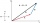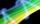# Vector - basic operations

There are given points A [-9; -2] B [2; 16] C [16; -2] and D [12; 18]

a. Determine the coordinates of the vectors u=AB v=CD s=DB
b. Calculate the sum of the vectors u + v
c. Calculate difference of vectors u-v
d. Determine the coordinates of the vector w = -7.u

Result

ux =  11
uy =  18
vx =  -4
vy =  20
sx =  10
sy =  2
(u+v)x =  7
(u+v)y =  38
(u-v)x =  15
(u-v)y =  -2
wx =  -77
wy =  -126

#### Solution:Leave us a comment of example and its solution (i.e. if it is still somewhat unclear...):Be the first to comment!#### To solve this example are needed these knowledge from mathematics:

For Basic calculations in analytic geometry is helpful line slope calculator. From coordinates of two points in the plane it calculate slope, normal and parametric line equation(s), slope, directional angle, direction vector, the length of segment, intersections the coordinate axes etc. Two vectors given by its magnitudes and by included angle can be added by our vector sum calculator.

## Next similar examples:Given that P = (5, 8) and Q = (6, 9), find the component form and magnitude of vector PQ.
2. Coordinates of vectorDetermine the coordinate of a vector u=CD if C(19;-7) and D(-16;-5)
3. VectorDetermine coordinates of the vector u=CD if C[19;-7], D[-16,-5].
4. VectorsFor vector w is true: w = 2u-5v. Determine coordinates of vector w if u=(3, -1), v=(12, -10)
5. LineLine p passing through A[-10, 6] and has direction vector v=(3, 2). Is point B[7, 30] on the line p?
6. BlocksThere are 9 interactive basic building blocks of an organization. How many two-blocks combinations are there?
7. Three workshopsThere are 2743 people working in three workshops. In the second workshop works 140 people more than in the first and in third works 4.2 times more than the second one. How many people work in each workshop?
8. Today in schoolThere are 9 girls and 11 boys in the class today. What is the probability that Suzan will go to the board today?
9. Theorem proveWe want to prove the sentence: If the natural number n is divisible by six, then n is divisible by three. From what assumption we started?
10. Reference angleFind the reference angle of each angle:
11. Three unknownsSolve the system of linear equations with three unknowns: A + B + C = 14 B - A - C = 4 2A - B + C = 0
12. TeamsHow many ways can divide 16 players into two teams of 8 member?
13. ChordsHow many 4-tones chords (chord = at the same time sounding different tones) is possible to play within 7 tones?
14. TrigonometryIs true equality? ?
15. LineIt is true that the lines that do not intersect are parallel?
16. PIN - codesHow many five-digit PIN - code can we create using the even numbers?
17. SequenceWrite the first 7 members of an arithmetic sequence: a1=-3, d=6.